Study Guide

# Definite Integrals - Average Value

## Average Value

We know how to take the average of a group of numbers: add all the numbers and divide by how many there are.

What would the "average value" of a function be?

A function like f (x) = x or f (x) = ex takes on infinitely many values. We can't add infinitely many values and divide by ∞.

However, there is a reasonable way to define the average value of a function on an interval.

First we're going to briefly revisit taking averages of numbers. We want to think about averages of numbers in a specific way that will make it easier to understand what the average of a function means.

• ### Averages with Numbers

To find the average of a bunch of numbers, we add all the numbers and divide by how many there are.

### Sample Problem

Five students took a test. Their scores were 60, 70, 80, 85, and 87. The average of their scores is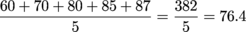In that example, if we add all the students' scores, we get 382. Now suppose we added up the students' scores and got 382, but that all the students got the same score on the test. In order for that to happen, each student would have had to score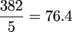We can think of averaging as redistributing points so that everyone gets an equal share.

### Sample Problem

Let f(x) be the function graphed below.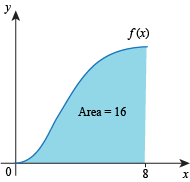We can see that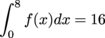Find a constant m such that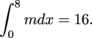In other words, find a constant m so that we have this: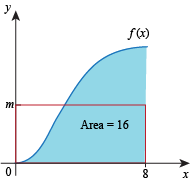Answer.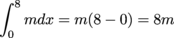. In order to have this equal 16, m must be 2.

We call this constant m the average value of f on [a, b]. When we take the integral of f on [a, b], we get some number. This number is like the sum of all the test scores: it's the accumulation of all the stuff.

To average that accumulation we give every x the same function value as every other x. Therefore we end up with a constant function whose integral on [a, b] is the same as the integral of f on [a, b].

The average value of f on [a, b] is a y-value. It's the particular y-value for which the weighted area between that y-value and the x-axis is equal to the integral of f on [a, b]. The average value of f on [a, b] is the (weighted) height of the rectangle whose (weighted) area is equal to the integral of f on [a, b].

Let f be non-negative for the sake of the pictures and let m be the average value of f on [a, b]. The area under m is a rectangle. Whatever area is in that rectangle but not under f must make up for the area that is under f but not part of the rectangle.